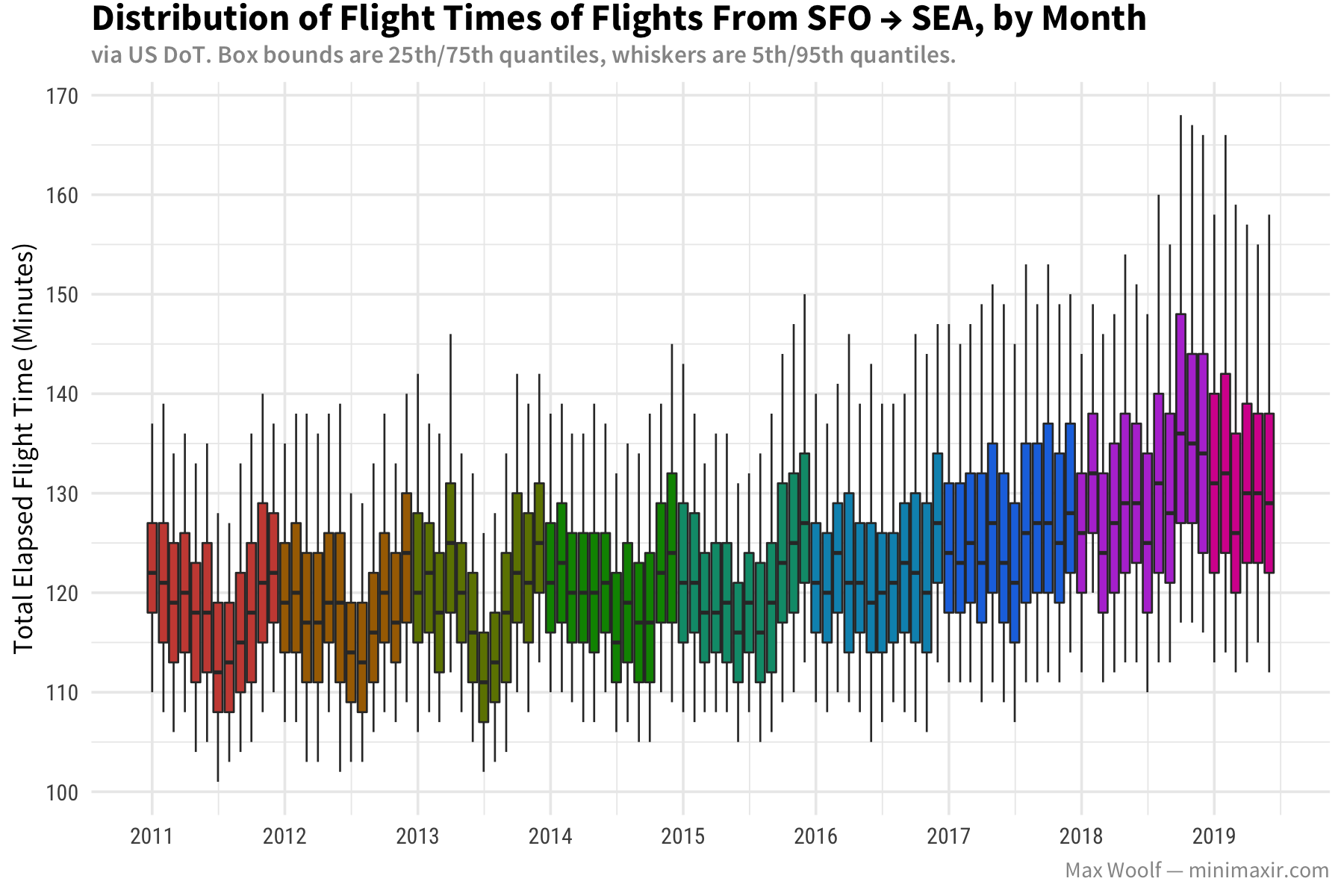This notebook is licensed under the MIT License. If you use the code or data visualization designs contained within this notebook, it would be greatly appreciated if proper attribution is given back to this notebook and/or myself. Thanks! :)

## 0.1 Setup

``library(tidyverse)``
``````Registered S3 method overwritten by 'dplyr':
method           from
print.rowwise_df
[37m── [1mAttaching packages[22m ──────────────────────────────────── tidyverse 1.2.1 ──[39m
[37m[32m✔[37m [34mggplot2[37m 3.2.1     [32m✔[37m [34mpurrr  [37m 0.3.2
[32m✔[37m [34mtibble [37m 2.1.3     [32m✔[37m [34mdplyr  [37m 0.8.3
[32m✔[37m [34mtidyr  [37m 1.0.0     [32m✔[37m [34mstringr[37m 1.4.0
[32m✔[37m [34mreadr  [37m 1.3.1     [32m✔[37m [34mforcats[37m 0.4.0[39m
[37m── [1mConflicts[22m ─────────────────────────────────────── tidyverse_conflicts() ──
``library(scales)``
``````
Attaching package: ‘scales’

The following object is masked from ‘package:purrr’:

col_factor``````
``library(lubridate)``
``````
Attaching package: ‘lubridate’

The following object is masked from ‘package:base’:

date``````
``````library(bigrquery)

sessionInfo()``````
``````R version 3.6.1 (2019-07-05)
Platform: x86_64-apple-darwin15.6.0 (64-bit)
Running under: macOS Catalina 10.15

Matrix products: default
BLAS:   /System/Library/Frameworks/Accelerate.framework/Versions/A/Frameworks/vecLib.framework/Versions/A/libBLAS.dylib
LAPACK: /Library/Frameworks/R.framework/Versions/3.6/Resources/lib/libRlapack.dylib

locale:
 en_US.UTF-8/en_US.UTF-8/en_US.UTF-8/C/en_US.UTF-8/en_US.UTF-8

attached base packages:
 stats     graphics  grDevices utils     datasets  methods   base

other attached packages:
 bigrquery_1.2.0 lubridate_1.7.4 scales_1.0.0    forcats_0.4.0
 tidyr_1.0.0     tibble_2.1.3    ggplot2_3.2.1   tidyverse_1.2.1

loaded via a namespace (and not attached):
 tidyselect_0.2.5 xfun_0.10        haven_2.1.1      lattice_0.20-38
 colorspace_1.4-1 vctrs_0.2.0      generics_0.0.2   htmltools_0.4.0
 yaml_2.2.0       base64enc_0.1-3  rlang_0.4.0      pillar_1.4.2
 glue_1.3.1       withr_2.1.2      DBI_1.0.0        bit64_0.9-7
 gtable_0.3.0     cellranger_1.1.0 rvest_0.3.4      evaluate_0.14
 knitr_1.25       broom_0.5.2      Rcpp_1.0.2       backports_1.1.5
 jsonlite_1.6     bit_1.1-14       hms_0.5.1        digest_0.6.21
 stringi_1.4.3    grid_3.6.1       cli_1.1.0        tools_3.6.1
 magrittr_1.5     lazyeval_0.2.2   crayon_1.3.4     pkgconfig_2.0.3
 zeallot_0.1.0    xml2_1.2.2       assertthat_0.2.1 rmarkdown_1.16
 httr_1.4.1       rstudioapi_0.10  R6_2.4.0         nlme_3.1-141
 compiler_3.6.1  ``````
``````# Switch to your own GCP project ID
project_id <- "<PROJECT_ID>"  ``````
``````theme_set(theme_minimal(base_size=9, base_family="Source Sans Pro") +
theme(plot.title = element_text(family="Source Sans Pro Bold", margin=margin(t = -0.1, b = 0.1, unit='cm'), size=12),
axis.title.x = element_text(),
axis.title.y = element_text(),
axis.text.y = element_text(family="Roboto Condensed"),
axis.text.x = element_text(family="Roboto Condensed"),
plot.subtitle = element_text(family="Source Sans Pro Semibold", color="#969696", size=8),
plot.caption = element_text(color="#969696"),
legend.title = element_text(),
legend.key.width = unit(0.25, unit='cm')))``````

# 1 EDA

## 1.1 SFO → SEA Flight Duration

``````query <- "
#standardSQL
SELECT Year, Month, num_flights,
time_q[OFFSET(5)] AS q_5,
time_q[OFFSET(25)] AS q_25,
time_q[OFFSET(50)] AS q_50,
time_q[OFFSET(75)] AS q_75,
time_q[OFFSET(95)] AS q_95
FROM (
SELECT Year, Month,
COUNT(*) as num_flights,
APPROX_QUANTILES(ActualElapsedTime, 100) AS time_q
FROM `fh-bigquery.flights.ontime_201908`
WHERE Origin = 'SFO'
AND Dest = 'SEA'
AND FlightDate_year > '2010-01-01'
GROUP BY Year, Month
)
ORDER BY Year, Month
"

``````Waiting for authentication in browser...
Press Esc/Ctrl + C to abort
Authentication complete.
Complete
Billed: 0 B
``````
Parsing [========================================================] ETA:  0s
``````
``````# ggplot2 likes a date for timeseries
df_tf <- df %>%
mutate(date = ymd(paste(Year, Month, "1")),
year_factor = factor(as.numeric(Year)))

NB: As of ggplot 3.2.0, you must have the `group` aesthetic for boxplots manually specifying the bounds. (https://stackoverflow.com/q/57192727)

``````plot <-
ggplot(df_tf,
aes(
x = date,
ymin = q_5,
lower = q_25,
middle = q_50,
upper = q_75,
ymax = q_95,
group = date,
fill = year_factor
)) +
geom_boxplot(stat = "identity", size=0.3) +
scale_fill_hue(l=50, guide=F) +
scale_x_date(date_breaks = '1 year', date_labels = "%Y") +
scale_y_continuous(breaks = pretty_breaks(6)) +
labs(
title = "Distribution of Flight Times of Flights From SFO → SEA, by Month",
subtitle = "via US DoT. Box bounds are 25th/75th quantiles, whiskers are 5th/95th quantiles.",
y = 'Total Elapsed Flight Time (Minutes)',
fill = '',
caption = 'Max Woolf — minimaxir.com'
) +
theme(axis.title.x = element_blank())

ggsave('sfo_sea_flight_duration.png',
plot,
width = 6,
height = 4)``````Alternate approach using ribbons (not used in final blog post since harder to visually parse, but present here for posterity):

``````plot <-
ggplot(df_tf,
aes(
x = date,
#group = "all",
color = year_factor,
fill = year_factor
)) +
geom_ribbon(aes(ymin = q_5, ymax = q_95), alpha=0.3, size=0, stat = "identity") +
geom_ribbon(aes(ymin = q_25, ymax = q_75), alpha=0.3, size=0, stat = "identity") +
geom_line(aes(y = q_50), stat = "identity") +
scale_color_hue(l=50, guide=F) +
scale_fill_hue(l=50, guide=F) +
scale_x_date(date_breaks = '1 year', date_labels = "%Y") +
scale_y_continuous(breaks = pretty_breaks(6)) +
labs(
title = "Distribution of Flight Times of Airline Flights From SFO → SEA, by Month",
subtitle = "via US DoT. Box bounds are 25th/75th quantiles, whiskers are 5th/95th quantiles",
y = 'Total Elapsed Flight Time (Minutes)',
fill = '',
color = '',
caption = 'Max Woolf — minimaxir.com'
) +
theme(axis.title.x = element_blank())

ggsave('sfo_sea_flight_duration_ribbon.png',
plot,
width = 6,
height = 4)``````